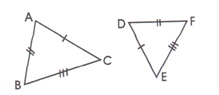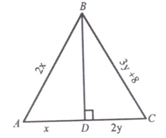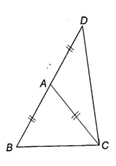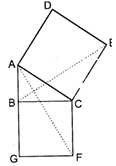# Worksheet for chapter-8 Triangle class 9

## Find CBSE Worksheet for chapter-8 Triangle class 9

CLASS-9

BOARD: CBSE

Mathematic Worksheet - 8

TOPIC: Triangle

For other CBSE Worksheet for class 9 Mathematic check out main page of Physics Wallah.

#### OBJECTIVE

1. ABCD is a square and P,Q,R are points on AB BC CD respectively such that AP=BQ=CR and ∠PQR=900 then ∠QPR
1. 45o
2. 50o
3. 60o
4. 70o
2. In a ΔPQR, PS is the bisector of ∠P and ∠Q=700,∠R=30o
1. QS>PQ>PR
2. QS<PQ<PR
3. PQ>QS>SR
4. PQ<QS<SR.
3. If D is the point on the side BC of a ΔABC then
4. none
4. For the given figure which one is correct:1. ΔABC ≅ΔDEF
2. ΔABC ≅ΔFED
3. ΔABC≌ΔDFE
4. ΔABC≌ΔEDF
5. In a right angled triangle One acute angle is double the other then the hypotenuse is
1. a) Equal to the smallest side
2. b) Double the smallest side
3. c) Triple the smallest side
4. d) None of these.

SUBJECTIVE

1. In the ΔABC given below
BD bisects ∠B and is perpendicular to AC .If the length of the sides of the triangle are expressed in terms of x and y as shown. Find the values of x and y.2. In the figure AB=AC=AD Prove that ∠BCD is a right angle4. In the figure ΔABC is a right angled triangle at B. ADEC and BCFG are squares prove that AF=BE5. ABCD is a square and EF||BD.E and F are the midpoint of BC and DC respectively. Prove that:
1. BE=DF

1. a
2. b
3. a
4. c
5. b

1. 1)16, 8